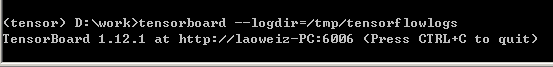## 2018/12/20

### TensorFlow 安裝與測試

17 TensorFlow 安裝與測試

⬛ 安裝 TensorFlow with Jupyter Notebook
TensorFlow 官網安裝說明 for Windows
https://www.tensorflow.org/install/pip

因先前安裝Anaconda時python的版本是3.7，但TensorFlow只支援到3.6，所以另建一個 Python 3.6 版的虛擬環境來執行 TensloFlow 。在Dos下輸入
> conda create -n tensorflow pip python=3.6

> pip install --ignore-installed --upgrade tensorflow

> pip install --ignore-installed --upgrade tensorflow-gpu

> pip install jupyter

> jupyter notebook 就會帶出notebook，這時在cell裡下
import sys
sys.version
# '3.6.6 | packaged by conda-forge | (default, Jul 26 2018, 11:48:23)
#   [MSC v.1900 64 bit (AMD64)]'


import tensorflow as tf
tf.__version__
# '1.12.0'


> pip install numpy scipy scikit-learn
pip pillow matplotlib panda keras

⬛ Hello Word
TensorFlow 和Keras是很常見到的deep learning (深度學習)的開發軟體，一般而言TensorFlow 比較低階，使用時需設定的細節較繁瑣，但功能較強大，而Keras 有點像是深度學習的套件，簡單幾行指令就可完成學習的模型。我們先由TensorFlow （ 以後討論有時縮寫成 tf ）開始，稍後再來探討 Keras。

tf和其他程式語言一個不同的地方是程式中分成兩大階段，第一階段是先設計系統的model (模型) ，這是要建立系統的graph (連接圖)，建好之後第二階是建立一個 Session (議程) 用以執行此連接圖並取得輸出。Tensor (張量)在物理及工程上是指用來表示純量、向量及其他張量之間的關係，但在程式語言裡就簡單的指如向量、矩陣等各種維度的量。flow是指流程，所以tf可以想像成很多張量所連接成的圖狀，而訊號在這些張量間流動。因而，可以想像在tf的連作方式是我們先把張量及其他元素連接好，再讓訊號流動，以達成深度學習的功能。

import tensorflow as tf
hello = tf.constant('Hello, TensorFlow!')
sess = tf.Session()
print(sess.run(hello))
sess.close()
# b'Hello, TensorFlow!'


import tensorflow as tf
a = tf.placeholder("float")
b = tf.placeholder("float")
mul_op = tf.multiply(a, b)
with tf.Session() as sess:
output= sess.run(output_array, feed_dict={a: 4, b: 2})
print(output)
# [[6.0, 8.0]]

tf 中用的變數種類除前述的constant外，還有Variable() class的物件，此種是真正的變數，使用時需給初值及tensor 的 shape (外形），在機器學習的過程中就是要調整這種變數的量，以達到系統最佳化，所以這種變數是可訓練的。另還有上面例子中使用的placeholder() ，可稱為持位器，就是宣告了一個變數在記憶體中的某個位置，所以變數的shape及data tpye要先指定，而真正的但就等到要用時再傳到那個位置。上例中a,b宣告成placeholder，形態是float，之後再進行a,b的相加及相乘，並把結果用一個$2\times 1$的array 來存放，這是此例的graph。接下來建立session物件，run()方法中指定output_array，但需用到a,b兩個持位器的變數，其值必需帶入，程式是以feed_dict帶入，所引進的變數是以python 的dict 形態傳進，關鍵字'feed_dict='可省略。程式最後印出結果。 tensorflow 變數可宣告成常數，或是placeholder，此變數只給定一變數型態（類似給定位址）但並未具有任何值。

⬛ Tensor and Variable

import tensorflow as tf
import numpy as np
arr_1d = np.array([1.3, 1, 4.0, 23.99])
print(arr_1d)
tensor_1d=tf.convert_to_tensor(arr_1d,dtype=tf.float64)
print(tensor_1d.shape)
print(tensor_1d.dtype)
with tf.Session() as sess:
print(sess.run(tensor_1d))
print(sess.run(tensor_1d))
print(sess.run(tensor_1d))

[ 1.3   1.    4.   23.99]
(4,)
<dtype: 'float64'>
[ 1.3   1.    4.   23.99]
1.3
4.0

import tensorflow as tf
sess = tf.InteractiveSession()
rv = tf.constant(((1,2,3),(4,5,6)))
print(rv.shape)
print(rv.eval())
sess.close()
#(2, 3)
#[[1 2 3]
# [4 5 6]]


import tensorflow as tf
sess = tf.InteractiveSession()
rv = tf.constant([[1,2,3],[4,5,6]])
print(rv.shape)
print(rv.eval())
sess.close()
#(2, 3)
#[[1 2 3]
# [4 5 6]]


import tensorflow as tf
sess = tf.InteractiveSession()
my_tensor = tf.random_normal((2,3))
print(my_tensor.shape)
print(my_tensor.eval())
sess.close()
#(2, 3)
#[[ 0.58983636 -0.3081955  -1.0226754 ]
# [ 0.9014508  -2.2361975  -1.6274402 ]]auto complete

tf.Variable(_)是要產生可以用來訓練的變數，如神經網路裡要用Backpropagation調整的係數，要宣告Variable物件時要給定初值，最常用的是給隨機值，一般可先產生個tensor，再把tensor的值當為Variable 的初值，如下程式
import tensorflow as tf
sess = tf.InteractiveSession()
tensor_1 = tf.random_normal((2,3))
my_var = tf.Variable(initial_value = tensor_1)
print(my_var)
sess.close()

<tf.Variable 'Variable_6:0' shape=(2, 3) dtype=float32_ref>

import tensorflow as tf
sess = tf.InteractiveSession()
tensor_1 = tf.random_normal((2,3))
my_var = tf.Variable(initial_value = tensor_1)
init = tf.global_variables_initializer()
sess.run(init)
print(my_var.eval())
sess.close()
#[-1.3067722   1.0955395  -0.8759342 ]
# [-0.01822465  0.4211547  -0.01513049]]


⬛ TensorBoard: (windows platform)
TensorBoard為一圖形介面工具，利於程式開發
import tensorflow as tf
a = tf.constant(10,name="a")
b = tf.constant(90,name="b")
y = tf.Variable(a+b*2, name="y")
model = tf.global_variables_initializer()
with tf.Session() as session:
merged = tf.summary.merge_all()
writer = tf.summary.FileWriter("/tmp/tensorflowlogs", session.graph)
session.run(model)
print(session.run(y))
#190


> tensorboard --logdir=/tmp/tensorflowlogstensorboard servertensorboard

> tensorboard --logdir=/tmp/tensorflowlogs --host=127.0.0.1127.0.0.1:6006